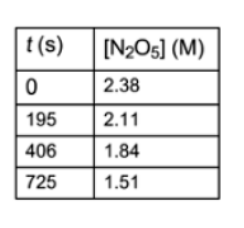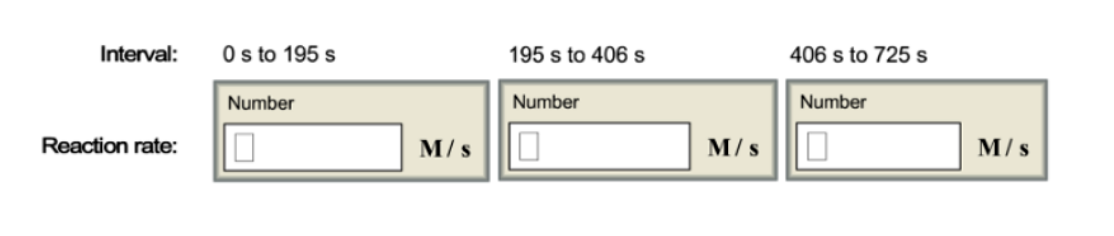# Problem: The decomposition of N2O5 can be described by the equation2N2O5 (soln) → 4NO2 (soln) + O2 (g)Given these data for the reaction at 45° C in carbon tetrachloride solution, calculate the average rate of reaction for each successive time interval.

🤓 Based on our data, we think this question is relevant for Professor Miao's class at CSUN.

###### FREE Expert Solution###### Problem Details

The decomposition of N2O5 can be described by the equation

2N2O5 (soln) → 4NO2 (soln) + O2 (g)

Given these data for the reaction at 45° C in carbon tetrachloride solution, calculate the average rate of reaction for each successive time interval.What scientific concept do you need to know in order to solve this problem?

Our tutors have indicated that to solve this problem you will need to apply the Average Rate of Reaction concept. You can view video lessons to learn Average Rate of Reaction. Or if you need more Average Rate of Reaction practice, you can also practice Average Rate of Reaction practice problems.

What is the difficulty of this problem?

Our tutors rated the difficulty ofThe decomposition of N2O5 can be described by the equation2N...as medium difficulty.

How long does this problem take to solve?

Our expert Chemistry tutor, Dasha took 5 minutes and 22 seconds to solve this problem. You can follow their steps in the video explanation above.

What professor is this problem relevant for?

Based on our data, we think this problem is relevant for Professor Miao's class at CSUN.